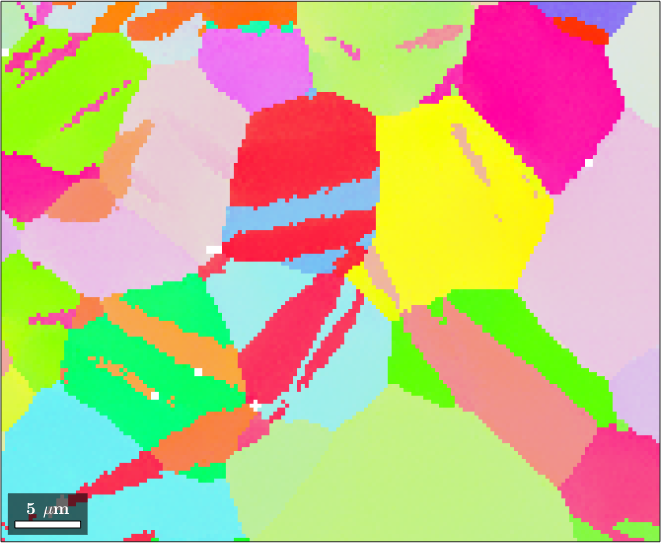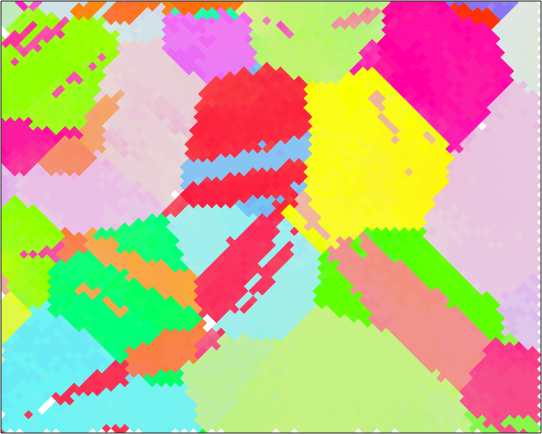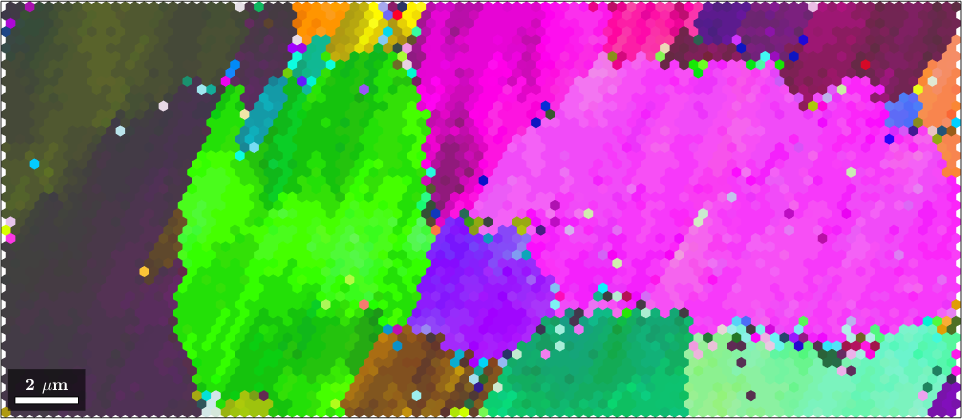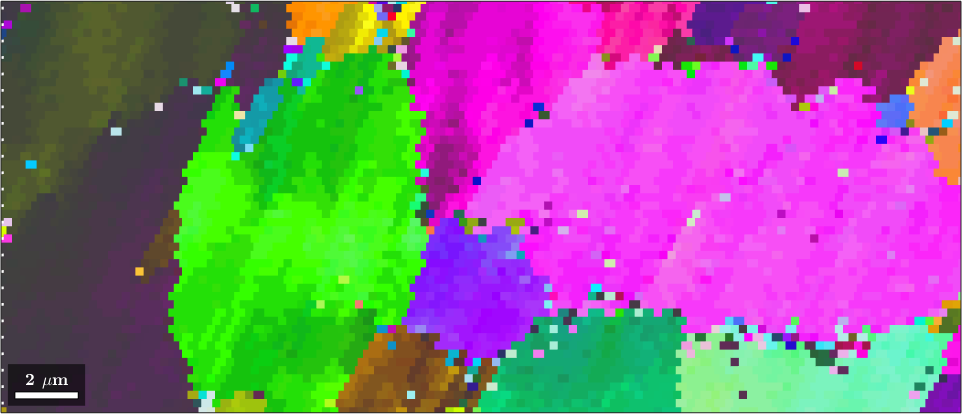Interpolating EBSD Data edit page

In the section Denoising and Filling Missing Data we have discussed how to work with noisy EBSD data the contained non indexed pixels. Hereby, we made the assumption that the grid before and after the operations is the same.

In this section we explain how to interpolate an EBSD map at positions that do not belong to the grid. Lets us consider a simple exampleNow we can use the command interp to interpolate the orientation at arbitrary coordinates x and y.

By default the command interp performs inverse distance interpolation. This is different to

which returns the nearest neighbour EBSD measurement. Lets have a look at the difference

## Change of the measurement grid

The command interp can be used to evaluate the EBSD map on a different grid, which might have higher or lower resolution or might even be rotated. Lets demonstrate thisNote, that we have not rotated the EBSD data but only the grid. All orientations as well as the position of all grains remains unchanged.

Another example is the change from a square to an hexagonal grid or vice versa. In this case the command interp is implicitely called by the command gridify. In order to demonstrate this functionality we start by EBSD data on a hex gridand resample the data on a square grid. To do so we first define a smaller square unit cell corresponding to the hexagonal unit cell